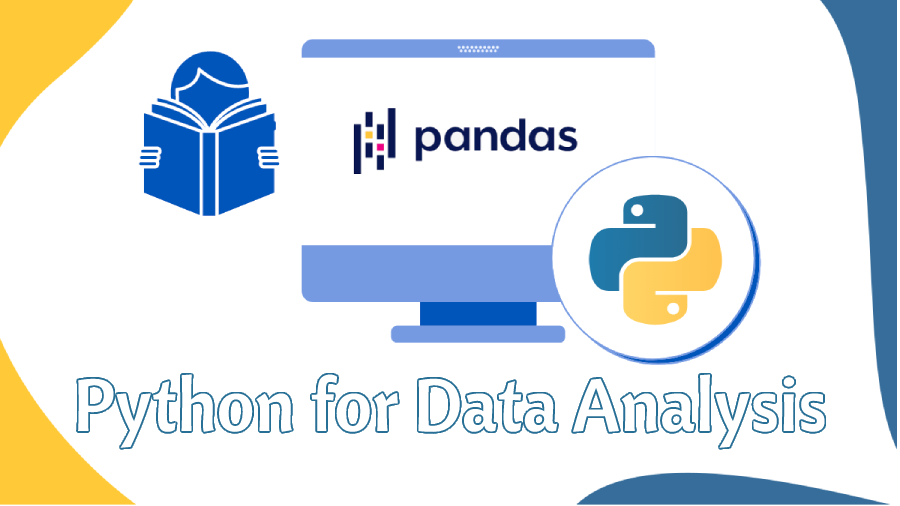• +91-9872993883
• +91-8283824812
• info@ris-ai.com

# Python Pandas Basic Concepts ¶

This guide is an introduction to the Data Analysis process using the Python . You will learn some of the most important pandas features for cleaningand visualizing from data.Pandas is an open-source library providing high performance, easy to use data structure and data analysis tools for python programming language. pandas is used in a wide range of fields including finance, economics, statics, analytics etc.

1. Less writting and more work done.
2. less time taken.
3. Easy to understand the data.
4. Easy to handle the large data.

1. The functionality becomes extremely confusing and they create a probleam for beginners.
2. Difficult syntax.

### Components of Pandas: Series and DataFrames¶

Series is a one-dimensional labelled array capable of holding data of any type(integer,string,float).

0    a
1    b
2    c
3    d
dtype: object

In :

### Pandas First Steps

Pandas is an to install. Open your terminal install it using either of the following commands

pip install pandas

import pandas as pd
import numpy as np
data = np.array(['a','b','c','d'])
s= pd.Series(data,index=[100,101,102,103])
print(s)

100    a
101    b
102    c
103    d
dtype: object

In :
import pandas as pd
import numpy as np
s=pd.Series(5,index=[0,1,2,3,4,5,6,7])
print(s)

0    5
1    5
2    5
3    5
4    5
5    5
6    5
7    5
dtype: int64


#### Data frame¶

A data frame is a two-dimensional data structure

In :
import pandas as pd
df= pd.DataFrame()
print(df)

Empty DataFrame
Columns: []
Index: []

In :
import pandas as pd
list= [1,2,3,4,5]
df= pd.DataFrame(list)
df

Out:
0
0 1
1 2
2 3
3 4
4 5
In :
import pandas as pd
data=[['ankita',21],['devshiri',21],['simran',21]]
df= pd.DataFrame(data,columns=['name','age'])
df

Out:
name age
0 ankita 21
1 devshiri 21
2 simran 21
In :
import pandas as pd
data=[['ankita',21],['devshiri',21],['simran',21]]
df= pd.DataFrame(data,columns=['name','age'],dtype=float)
df

Out:
name age
0 ankita 21.0
1 devshiri 21.0
2 simran 21.0
In :
a=np.reshape(np.arange(1,801),(200,4))
b=np.reshape(np.arange(1,601),(200,3))
c=np.concatenate((a,b),axis=1)
df =pd.DataFrame(c)
df

Out:
0 1 2 3 4 5 6
0 1 2 3 4 1 2 3
1 5 6 7 8 4 5 6
2 9 10 11 12 7 8 9
3 13 14 15 16 10 11 12
4 17 18 19 20 13 14 15
... ... ... ... ... ... ... ...
195 781 782 783 784 586 587 588
196 785 786 787 788 589 590 591
197 789 790 791 792 592 593 594
198 793 794 795 796 595 596 597
199 797 798 799 800 598 599 600

200 rows × 7 columns

# Conclusion-¶

You've successfully completed the Pandas Tutorial for Dataframe and Series with code examples.